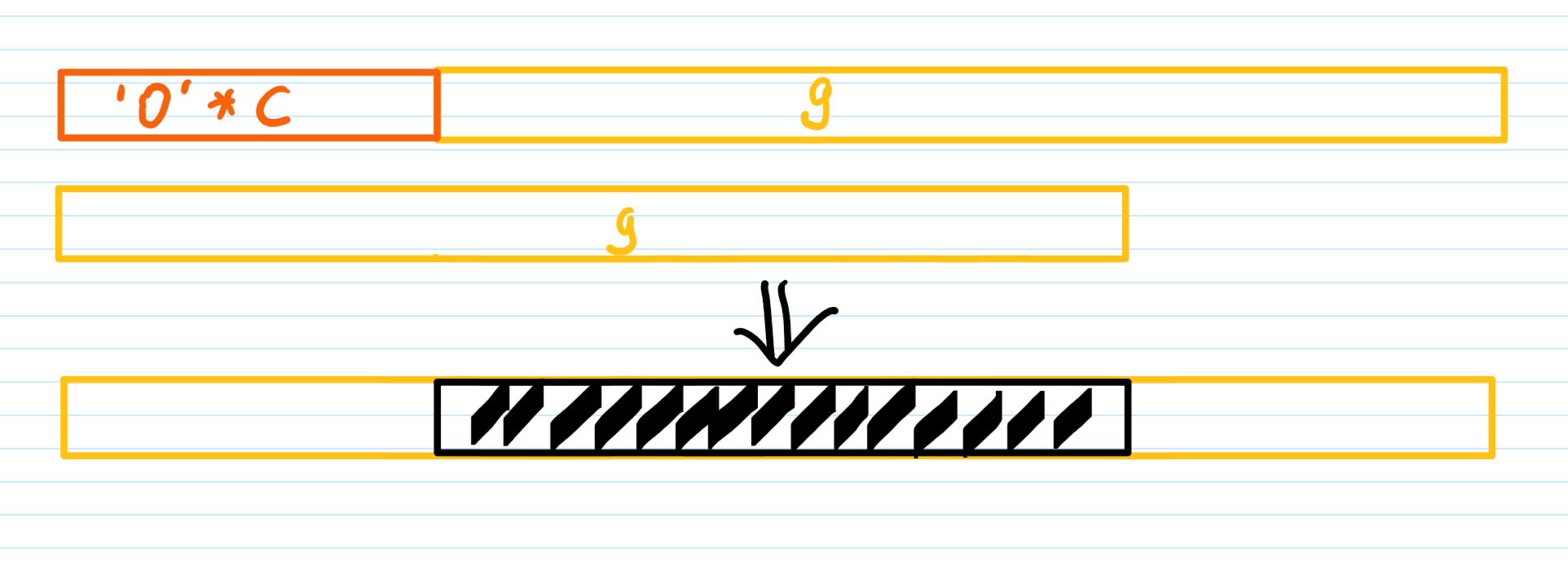# 2021 CryptoCTF

POINTS：41

exp：

## Keybase

POINTS：481. 首先我们发送两组共32字节的明文 “A”*32 过去，会得到14字节的密钥和一组模糊密文和一组清晰密文
2. 我们爆破密钥的剩余两个字节，然后用爆破出来的密钥尝试对第二组密文进行解密，解密后的结果和第一组模糊密文中的清晰部分进行异或，如果得到的均为”A“，则我们的密钥爆破正确。
3. 由于CBC模式，我们还需要iv。此时我们拥有密钥，明文；如果拥有第一组密文，我们对第一组密文进行解密并与明文异或即可知道iv
4. 需要意识到的是，第一组密文用于第二组明文加密，而第二组明文我们又是知道的，所以我们使用密钥解密第二组密文，并与第二组明文异或得到第一组密文，然后解密第一组密文，与第一组明文异或，得到iv
5. 使用密钥和iv解密flag的密文，获取flag

## Rima

POINTS:56类似这样两个数组相加。最后还将当前得到的值拼接，视为五进制

1. 首先我们爆破flag的长度，我们知道密文的长度为 a * f + c 和 b * f + c，而a ,b,c 又和f直接相关，所以这很好判断。

2. 然后我们先恢复这个”移位C“的向量加，以上图为例，由于最后结果仍然保留了原来g数组的开头和结尾，故中间部分是很好恢复的。

3. 最后我们在恢复一个”移位1“的向量加即可解出flag。

（其实用不到两个enc，有一个就够了。）

exp.py

## Titu

POINTS：69

$(x^2+1)\cdot(y^2+1)-2\cdot(x-y)(xy-1) - 4xy = ((x+1)(y+1))^2$

$k=(2\cdot 3\cdot 11^2\cdot 19\cdot 47\cdot 71\cdot 3449\cdot 11953\cdot 5485619\cdot 2035395403834744453 \cdot 17258104558019725087 \cdot 1357459302115148222329561139218955500171643099)^2$

## Symbols

POINTS：70

h, my eyes, my eyes! People still can solve this kind of cryptography? Mathematicians should love this one!$\Cap \Cap \Theta \Finv { \Pi \ltimes \aleph y _ \wp \infty \therefore \heartsuit _ \Lsh \aleph \Theta \eth \Xi }$

CCTF{Play_with_LaTeX}

POINTS：71

transpose函数为矩阵转置

vsum函数为向量加法

sprod函数为向量的倍增

## Salt and Pepper

POINTS：71

./hash_extender -f md5 -d -s 5f72c4360a2287bc269e0ccba6fc24ba -a n3T4Dm1n -l 19

得到

得到

3. 那么我们以

用户名：\x80\x00\x00\x00\x00\x00\x00\x00\x00\x00\x00\x00\x00\x00\x00\x00\x00\x00\x00\x00\x00\x00\x00\x00\x00\x00\x00\x00\x00\x00\x00\x00\x00\x00\x00\x00\x00\x98\x00\x00\x00\x00\x00\x00\x00n3T4Dm1n

密码：\x80\x00\x00\x00\x00\x00\x00\x00\x00\x00\x00\x00\x00\x00\x00\x00\x00\x00\x00\x00\x00\x00\x00\x00\x00\x00\x00\x00\x00\x00\x00\x00\x00\x00\x00\x00\x00\x00\x00\x00\x00\x00\x00\x00\x98P4s5W0rd

哈希：

83875efbe020ced3e2c5ecc908edc98481eba47f

作为输入即可通过验证，获取flag

## Hamul

POINTS：83

emmm，RSA经典整活，就是分解特殊构造的n。

$PP = P \cdot 10^{x’} + Q = (p\cdot 10^{x} +q)\cdot 10^{x’} + (q\cdot 10^{y} +p)=p\cdot (10^{x+x’})+q\cdot (10^{x’}+10^{y})+p$

$QQ = Q \cdot 10^{y’} + P = (q\cdot 10^{y} +p)\cdot 10^{y’} + (p\cdot 10^{x} +q) =q \cdot(10^{y+y’}) + p \cdot(10^y+10^{y’})+q$

$N = PP \cdot QQ = pq\cdot10^{x+x’+y+y’} + \cdots+p\cdot q$

POINTS：91

exp.py

## Onlude

POINTS：95

R 和 S 是一个随机矩阵，

L，U 分别是 S的下三角矩阵和上三角矩阵，会有 $S==L \times U$

$E = L^{-1} \times Y = L^{-1} \times S \times X$

$E = L^{-1} \times L \times U \times(A+R)$

$E = U \times A + U \times R$

$S^8 = (L \times U)^8 = (L \times U \times L \times U)^4$

## Maid

POINTS：119

e:使用公钥加密 $c \equiv msg^2 \pmod n$

d:使用私钥解密 未知

s:给出flag的密文 $c \equiv m^{65537} \pmod n$

$n$ 也没给，不过这好搞，用两次加密功能，然后 $gcd(m_1^2-c_1,m_2^2-c_2)$ 就好了。

（这里后面放出源码了，就模拟一下当时的场景好了。）

$m_p \equiv c^{(p+1)/4} \pmod p$

$i=(c-m_p^2)//p$

$j \equiv \frac{i} {2 \cdot m_p} \pmod p$

$m = m_p + j \cdot p$

（不难知道 $c \equiv m^2 \pmod p$，$c \equiv m^2 \pmod {p^2}$，$c \equiv m^2 \pmod q$ ）

$m_p \equiv (m^2)^{(p+1)/4} \equiv m^{(p+1)/2} \equiv m \cdot m^{(p-1)/2}\equiv \pm m \pmod p$

$i = (m^2 - m_p^2 ) // p = (m^2 + k_1p^2q - m^2 - k_2p)//p=k_1pq-k_2$

$j \equiv \frac{i}{2m_p} \equiv \frac{k_1pq-k_2}{2m_p} \equiv \frac{-k_2}{2m_p}\pmod p$

$m = m_p + j \cdot p = m_p + \frac{-k_2p}{2m_p}$ 这一步似乎是想得到 $m \pmod {p^2}$ ？

$m_p \equiv (-1)^{(p+1)/4} \equiv 1 \ or -1\pmod p$

$i = (-1 - 1)// p = -1$

$j \equiv -2^{-1} \pmod p$

$m = 1 +j \cdot p$

$i = (-1 - 1- k_1p)// p = -k_1$

$j \equiv \frac{k_1}{2}\pmod p$

$m = -1 + j \cdot p$ (然而好像触发 $m \ge \frac{p^2}{2},return \ p^2 -m,$，所以得到 $m = 1-j\cdot p +p^2$)

## Wolf

PONITS：128

emmm，那就暴力连接，当niv=1的时候，再爆破一下niv的值进行解密，一共也就256种可能。

## Ferman

POINTS:134

（PS：对于方程$x^2+y^2=z^7$，我们利用高斯分解似乎能够找到很多组满足条件的解 $x,y$，取决于高斯分解 $z$ 后它能有多少个因子）

## RSAphantine

POINTS:142

1. $2z^5 - x^3 + yz=a$

2. $x^4+y^5+xyz=b$

3. $y^6+2z^5+zy=c$

$y^6+x^3=c-a$

（感觉出题人处理的比较好，c-a只有两个因子）那么显然，这里有 $y^2+x=3133713317731333$

## FROZEN

POINTS: 142

$p$ 是一个约为 30bit 的素数

exp

PS：这道题的flag是 CCTF{Lattice_bA5eD_T3cHn1QuE_70_Br34K_LCG!!}，看来作者原意应该是让我们有lattice去恢复未知位，不过这里通过已知明文攻击能够获取到的低位太多了，这里需要再稍微调整一下。

## LINDA

POINTS：169

$$cc \equiv m \cdot w^{r+s} \pmod p \ cb \equiv v^s \pmod p \ ca \equiv u^r \pmod p$$

exp

PS：这道题能有这么高的分倒是有点不可思议，可能大部分选手没发现 $p-1$ 是光滑的叭。

POINTS：217

## ELEGANT CURVE

POINTS：217

flag: CCTF{Pl4yIn9_Wi7H_ECC_1Z_liK3_pLAiNg_wiTh_Fir3!!}

PS：感觉这两道题也可以控制参数使得两条曲线的阶都是比较光滑的，然后再解离散对数？

## DOUBLE MIFF

POINTS：217

$$x_0 \equiv \frac{(x_2+x_3)\cdot(1+y_2y_3)}{(1+x_2x_3)(1-y_2y_3)} \pmod p$$

$$x_0 \equiv \frac{2x_1(1+y_1^2)}{(1+x_1^2)(1-y_1^2)} \pmod p$$

$$(x_2+x_3)\cdot(1+y_2y_3) \cdot (1+x_1^2)(1-y_1^2)-2x_1(1+y_1^2)\cdot(1+x_2x_3)(1-y_2y_3) \equiv 0 \pmod p$$

$$(y_2+y_3)\cdot(1+x_2x_3) \cdot (1+y_1^2)(1-x_1^2)-2y_1(1+x_1^2)\cdot(1+y_2y_3)(1-x_2x_3) \equiv 0 \pmod p$$

$$k \equiv \frac{a}{b} \equiv \frac{y(x^2-1)}{x(y^2-1)}$$

$$k\frac{x}{x^2-1} \equiv \frac{y}{y^2-1}$$

$$x_3 \equiv \frac{2x_4(1+y_4^2)}{(1+x_4^2)(1-y_4^2)} \pmod p$$

$$y_3 \equiv \frac{2y_4(1+x_4^2)}{(1+y_4^2)(1-x_4^2)} \pmod p$$

$$x_3y_3 \equiv \frac{4x_4y_4}{(x_4^2-1)(y_4^2-1)} \equiv 4k(\frac{x_4}{x_4^2-1})^2 \pmod p$$

$$(\frac{x_4^2-1}{x_4})^2 \equiv \frac{4k}{x_3y_3} \pmod p$$

## ECCHIMERA

POINTS：271

n可以分解

kp=p，smart attack

sage: factor(kq)
2^4 * 3 * 13 * 233 * 4253 * 49555349 * 7418313402470596923151

flag:CCTF{m1X3d_VeR5!0n_oF_3Cc!}

## ROHALD

POINTS：180

$X_1 = x_1^2 +y_1^2-c^2(1+dx_1^2y_1^2) = k_1p$

$X_2 = x_2^2 +y_2^2-c^2(1+dx_2^2y_2^2) = k_2p$

$E_{c,d} : u^2 + v^2 -c^2 (1+du^2v^2)=0$

$u_{P+Q} = \frac{(u_Pv_Q)+(v_Pu_Q)}{c(1+du_Pu_Qv_Pv_Q)}$

$v_{P+Q}=\frac{(-u_Pv_Q)+(v_Pu_Q)}{c(1-du_Pu_Qv_Pv_Q)}$

Edwards Curve to $u’^2 + v’^2 =1+d’u’^2v’^2$ $(d’=dc^4,u’ = \frac{u}{c},v’=\frac{v}{c})$

to Montgomery $By’^2=x’^3+Ax’^2+x$ $(x’=\frac{1+v’}{1-v’},y’=\frac{2(1+v’)}{u’(1-v’)},A=\frac{2(1+d’)}{1-d’},B = \frac{1}{1-d’})$

to Weierstrass $y^2 =x^3 +ax+b$ $(x = \frac{x’}{B}+\frac{A}{3B},y=\frac{y’}{B},a=\frac{3-A^2}{3B^2},b=\frac{2A^3-9A}{27B^3})$

POINTS：194

## TRUNC

POINTS：334

CCTF{__ECC_Bi4seD_N0nCE_53ns3_LLL!!!}

（PS：不过看着flag，估计要构造格，又非预期了，，，）

POINTS：477

（PS：

## DORSA

POINTS：450

$$p=uv+1,q=vy+1,r=xy+1,s=kv+1，\phi=(p-1)(r-1)=uvxy,ed\equiv 1\pmod \phi,k=(ed-1)/\phi$$

$$\frac{n_2}{n_1} = \frac{qs}{pr}=\frac{(uy+1)(kv+1)}{(uv+1)(xy+1)}\approx\frac{uykv}{uvxy}=\frac{k}{x}$$

（这里假设 $gcd(k,x)=1$ 了，但是也不一定是 1 叭，但也应该不大，可以小爆破一下？）

$$k\phi+1 =ed\equiv 0 \pmod e \to \phi \equiv -1 \cdot k^{-1} \pmod e$$

$$\phi =uvxy \equiv 0 \pmod x$$

$$| \phi -n_1|=|(p-1)(r-1)-pr| \approx p+q \le 2^{513}$$

(PS：看这flag，又非预期了似乎。。。。)

## POLISH

POINTS：477

$$x^2(y-a)+y^2(x-a)=b$$

$$xy(y+x)-a(x^2-y^2)=b$$

$$uv-a(u^2-2v)=b$$

$$(u+2a)v=au^2+b$$

$$v=\frac{au^2+b}{u+2a}$$

$$v=\frac{au^2+b+2a^2u-2a^2u}{u+2a}=au+\frac{b-2a^2u-4a^3+4a^3}{u+2a}=au-2a^2+\frac{b+4a^3}{u+2a}$$

$$b+4a^3=n$$

（PS：由于e很大，所以个人PC挺难跑的，这里解题者是使用了20cores的计算机多线程去跑的。。。。）

flag:CCTF{Par7ial_K3y_Exp0sure_At7ack_0n_L0w_3xP_RSA}

（PS：看这flag，$e$ 也不小啊，是不是放错了，，，，，）

×